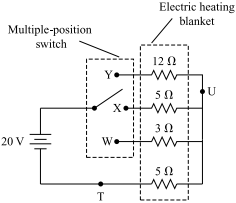Massachusetts Comprehensive Assessment System

# 2015, High School Intro Physics

Reporting Category: Electromagnetism
Standard: 5.3 - Analyze simple arrangements of electrical components in both series and parallel circuits. Recognize symbols and understand the functions of common circuit elements (battery, connecting wire, switch, fuse, resistance) in a schematic diagram.

The diagram below shows a simplified schematic for an adjustable electric heating blanket. The switch may be connected to point W, X, or Y depending on the desired level of warmth.1. Calculate the current generated in the blanket when the switch is connected to point X. Show your calculations and include units in your answer.
1. Calculate the power generated in the blanket when the switch is connected to point X. Show your calculations and include units in your answer.
1. Explain why a fuse at point T would be more effective in this circuit than a fuse at point U.
1. Identify which point the switch should be connected to in order to generate the most warmth. Explain your answer.

## Scoring Guide and Sample Student Work

Select a score point in the table below to view the sample student response.

ScoreDescription
4 The response demonstrates a thorough understanding of current, power, and the arrangements of electrical components in series circuits. The response correctly calculates the current and power generated by the blanket when the switch is connected to point X. The response also clearly explains why a fuse at point T would be more effective than a fuse at point U, and correctly identifies and clearly explains which switch point will generate the most warmth.
4
3 The response demonstrates a general understanding of current, power, and the arrangements of electrical components in series circuits.
2 The response demonstrates a limited understanding of current, power, and the arrangements of electrical components in series circuits.
1 The response demonstrates a minimal understanding of current, power, and the arrangements of electrical components in series circuits.
0 The response is incorrect or contains some correct work that is irrelevant to the skill or concept being measured.
Note: There are 2 sample student responses for Score Point 4.

## High School Intro Physics

Question 12: Electromagnetism
Question 23: Motion and Forces
Question 32: Motion and Forces
Question 44: Heat and Heat Transfer

Last Updated: April 13, 2020